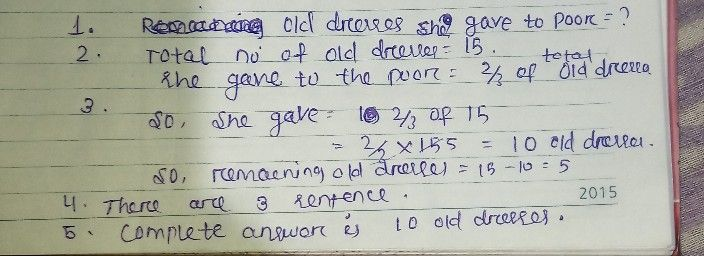Symbol
ProblemSheila has $15$ old dresses. If she gave $2/3$ of them to the poor, how many old dresses did she give? $1.$ What is asked? $2$ What are the given facts?. $3$ What operation to be used? $4$ What is the number sentence? $5$ What is the complete answer. Write the solution.
1st-6th grade
Other
Search count: 112
SolutionQanda teacher - priyamamun